EENS 2110 Mineralogy Tulane University Prof. Stephen A. Nelson Crystallographic Calculations

 Crystallographic Calculations Crystallographic calculations involve the following: Miller Indices (hkl) Axial ratios  - . a: 1: c ρ and φ angles corresponding to a Miller Index (hkl) angles between crystallographic axes if in monoclinic, or triclinic systems. Most of what you will do in lab or on exams will involve crystallographic calculations in the orthorhombic, tetragonal, hexagonal, or isometric systems, where the axes angles are fixed. Note that you will always be given enough information to solve the problem. Some of the problems you might be expected to solve involve determination of Miller Indices from the ρ and φ angles, to determine the ρ and φ angles for faces of mineral with known axial ratios, or to determine axial ratios of minerals that have faces of known ρ and φ angles.   First let's review some of the things we know about Miller indices and ρ and φ angles. In the orthorhombic, tetragonal, or isometric systems. If h is 0, i.e. (0kl) is the Miller Index, then the face is parallel to the a crystallographic axis. So, φ = 0o or φ = 180o         If k is 0 i.e. (h0l) is the Miller Index, then the face is parallel to the b crystallographic axis. So, φ = 90o or φ = 270o         If l is 0, i.e. (hk0) is the Miller Index, then the face is parallel to the c crystallographic axis. So, ρ = 90o If h & k are 0, i.e. (00l) is the Miller Index, then the face is perpendicular to the c axis So, ρ = 0o or ρ = 180o, and φ is undefined. If h & l are 0, i.e. (0k0) is the Miller Index, then the face is perpendicular to the b axis. So, ρ = 90o and φ = 0o or φ = 180o.         If l and k are 0, i.e. (h00) is the Miller Index, then the face is perpendicular to the a axis. So, ρ = 90o and φ = 90o or φ = 270o. Now let's do some examples
 Given Miller indices and ρ and φ angles for crystal faces that, in combination, intersect all crystallographic axes, calculate the axial ratios of the mineral. Faces         ρ          φ (110)       90o      45o (011)       70o        0o
 For the (110) we note that it does not intersect the c axis, so we can look at it in the two dimensional plane containing the a and b axes, as shown in the drawing below. From this face we should be able to determine the a/b axial ratio.  Since the φ angle is the angle between the normal to the face and the b axis, by similar triangles we know that the φ angle also occurs between the a axis and the face.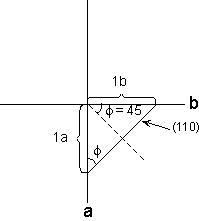Thus, we can write:   tan 45o = 1b/1a then         1a/1b = 1/tan 45o= 1           So, a/b: b/b = 1: 1
 We next note that the (011) intersects the b and c axes only, so we can examine this face in the plane containing only b and c, as shown below.  From this drawing we can obtain the c/b axial ratio.  Since the ρ angle is the angle between the pole to the face and the c axis, again by similar triangles we know that the ρ angle also occurs between  the b axis and the (011) face.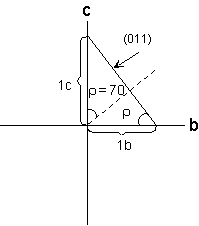Thus, we can write:   tan 70o = 1c/1b then         c/b = arctan 70o           So, c/b = 2.7475 so, a : b : c = 1 : 1 : 2.7475 and the mineral must be tetragonal, since a/b =1.
 b.    Faces     ρ       φ       (120)      90o    70o       (011)      32o      0o The face (120) does not intersect the c axis, so we can look at this face in the plane containing only the a and b axes.  We must also remember that Miller Indices represent the inverse of the intercepts, so the face (120) intersects the a axis at twice the number of unit lengths that it intersects the b axis. Since the φ angle is the angle between the normal to the face and the b axis, by similar triangles we know that the φ angle also occurs between the a axis and the (120) face.Then we can write: tan 70o = 1b/2a           a/b = 1/2tan70o 1a/1b = 0.18199
 We next note that the (011) intersects the b and c axes only, so we can examine this face in the plane containing only b and c, as shown below.  From this drawing we can obtain the c/b axial ratio.  Since the ρ angle is the angle between the face and the c axis, again by similar triangles we know that the ρ angle also occurs between  the b axis and the (011) face.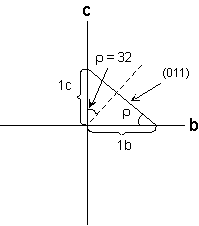For this face we can determine that   tan 32o = 1c/1b     1c/1b = 0.6248 so, a : b : c =  0.18199 : 1 : 0.6248, and the mineral is orthorhombic
 c.       Faces    ρ     φ          (311)    24o  33o This face is more complicated because it intersects all three axes.
 We first attempt to draw a 3-dimensional view of this face.  Notice that the φ angle is measured in the horizontal plane that includes the a and b axes. The ρ angle is measured in a vertical plane that includes the c axis and the line normal to the face in the a - b horizontal plane, and is measured between the c axis and a line normal to the face. Note also, that for the (311) face, the intercept on the a axis is 1/3 what it is on the b and c axes, because the Miller Index is the inverse of the intercepts..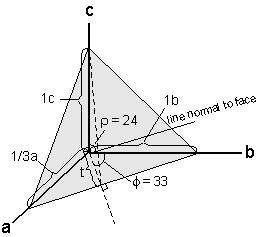We can determine the a/b part of the axial ratio by looking at the projection of this face in the a - b plane. 1b/(1/3)a = tan 33o 1a/3b = 1/tan 33 1a/1b = 3/tan 33 1a/1b = 4.6196
 In order to determine the length of the c axis, we need to know the length of the line labeled t, because this line forms the base of the triangle in which the ρ angle is measured.  The length of the line t is:         t/b = cos 33o t/b = 0.8397 t =  0.8397 b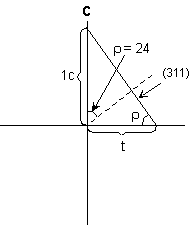We can now use this to determine the c/b axial ratio.  1c/t = tan 24o 1c = 0.8387 b tan 24o c/b = 0.3747 Thus, the axial ratio for this mineral is 4.6196 : 1 : 0.3747

 Now we'll look at an example where we are given the axial ratio of the mineral and asked to calculate the ρ and φ angles for the faces.   Given the axial ratio for a mineral is 1 : 1 : 5.0, what are ρ and φ for the face (111).
 We first attempt to draw a 3-dimensional view of this face.  Notice that again the φ angle is measured in the horizontal plane that includes the a and b axes. The ρ angle is measured in a vertical plane that includes the c axis and the line normal to the face in the a - b horizontal plane, and is measured between the c axis and a line normal to the face. In this case the intercept on the all three axes is 1.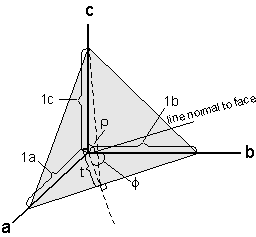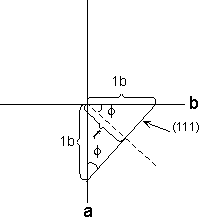Since the φ angle for this face is measured in the horizontal a - b plane, we can draw the plane containing only the a and b axes to determine the angle.  Since the axial ratio tells us that the relative lengths of the a and b axes are equal  tan φ = 1b/1b = 1 φ = 45o

 In order to determine the ρ angle, we need to know the length of the line labeled t, because this line forms the base of the triangle in which the ρ angle is measured.  The length of the line t is:                 t/b = cos 45o t = 0.7071 bNow we can determine the  angle by drawing the plane that includes the c axis and the line t.  In this plane we can let the length of the c = 5b, from the axial ratio.  Then: tan ρ = 5b/t tan ρ = 5b/0.7071b tan ρ = 5/0.7071 tan ρ = 7.071 ρ = arctan (7.071) = 81.95o So for the (111) face in this crystal ρ =81.95o and φ = 45o.

 Given the tetragonal crystal shown below and the following information:   For the face (101) ρ = 70o φ = 90o What is the axial ratio for this crystal? What are the Miller Indices for the faces labeled (0kl) and (h0l), given that both of these faces have ρ = 53.9478o?To find the axial ratio we note that we can use the face (101) and draw this in plane of the a and c axes (since the face doesn't intersect the b axis. Then, we can determine that    tan ρ = 1c/1a so, tan 70o = c/a = 2.7475 and the axial ratio is:  1 :1 : 2.7475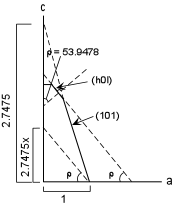To find the Miller indices of the face (h0l) we proceed as follows: Since all we need is the relative lengths of intersection to calculate the parameters, we can assume one of the lengths = 1, i.e. 1a. This is the same as moving the face parallel to itself so that it intersects the a axis at a unit length of 1. The face intersects the c axis at 2.7475x, where the 2.7475 value is the length of the c axis relative to the unit length of the a axis.  Then   tan (53.9478) = 2.7475x/1, and thus, x = 0.5 The parameters for this face are then: 1, ¥, 1/2, which can be inverted to give the Miller Index -  (102).
 Since the mineral is tetragonal, the face labeled (0hl) would have the Miller Index (012). Problems such as these could be asked on any laboratory exam. Return to EENS 2110 Page